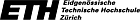Java and the Java Virtual Machine (R. Stärk, J. Schmid, E. Börger)

# Some legal Java programs are not bytecode verifiable

There are Java programs which are not accepted by most of the well known verifiers. The Jbook explains why the following two valid Java programs are and as a matter of fact should be rejected by most known verifiers. Jbook restricts the class of valid Java programs in such a way that all programs can indeed be verified.

The examples programs below which are rejected by most bytecode verifiers (including JDK 1.2, JDK 1.3, Netscape 4.73-4.77, Microsoft VM for Java 5.0 and 5.5, and the Kimera Verifier) are accepted by Bernard Serpette's bytecode verifier. Serpette's bytecode verifier assigns more than one type frame to an instruction. It verifies a subroutine several times, once for each call of the subroutine.

Sun's J2SE 1.4.2 compiler inlines the code of the finally block and does not use jsr/ret. Therefore, the examples in Figures 16.8 and 16.9 are accepted by the bytecode verifier when compiled under 1.4.2.

Figure 16.8 in the Jbook shows the following valid Java program:
 ```public class Figure16_8 { public Figure16_8() { m(false); } static int m(boolean b) { int i; try { if (b) return 1; i = 2; } finally { if (b) i = 3; } return i; } } ```
This Java program cannot be verified by most known verifiers. In the following lines you can see whether your browser accepts or rejects the bytecode of the above Java program. If there is no output below, check whether Java is enabled in your browser.

Figure 16.9 in the Jbook shows the following valid Java program:
 ```public class Figure16_9 { public Figure16_9() { m(true); } static int m(boolean b) { int i; L: { try { if (b) return 1; i = 2; if (b) break L; } finally { if (b) i = 3; } i = 4; } return i; } } ```
This Java program cannot be verified by most known verifiers. In the following lines you can see whether your browser accepts or rejects the bytecode of the above Java program. If there is no output below, check whether Java is enabled in your browser.

Home

 ETH Home March 2001 Responsible